# A Survival Model in Stan

#### 2018-11-15

The goal of this short case study is two-fold. Firstly, I wish to demonstrate essentials of a Bayesian workflow using the probabilistic programming language Stan. Secondly the analysis shows that doing posterior (and prior) predictive checks for (right) censored (survival) data requires an adjustment of the standard method utilized for drawing samples from the posterior preditive distribution.

The dataset we consider here is known as the mastectomy dataset1 This case study is motivated by Austin Rochford’s related PyMC3 blog post. See also this updated version, now part of the official PyMC3 documentation. The same dataset was also studied by the same author in this related case study.. As the title already suggest, we will implement the simplest, and probably most commonly used survival model, also sometimes known as (Cox’s) proportional hazard model.

The beauty or advantage of the Bayesian framework is that we can avoid any technicality or approximation due to what is known as ties in the dataset, usually encountered in real datasets and which need special treatments/considerations in the frequentist Cox Proportional Hazard framework (see e.g. coxph). This is because we model the baseline hazard explicitly and hence do not need to revert to what is known as a pseudo-likelihood2 For a good and concise description of the frequentist approach and the utilized pseudo-likelihood, see chapter 9.4 and the related appendix in Computer Age Statistical Inference by Efron and Hastie..

Moreover, the Bayesian framework allows us to easily scrutinize our model(s) beyond what is apparently possible with standard frequentist approaches, hence allowing us to quickly identify aspects of the true/observed dataset that are not well described by our model(s). This allows us to systematically and iteratively probe the applicability and limits of our model(s).

Before we dive into the matter, I would like to thank Tiago Cabaço and Jacob de Zoete for valuable feedback on an early draft of this case study. I also would like to thank Aki Vehtari, Arya Pourzanjani and Jacki Novik for fruitful discussions on survival models, which will find applications beyond this case study… Stay tuned.

Now, let’s first have a look at the data:

A mastectomy dataset

time event metastized
23 TRUE 0
47 TRUE 0
69 TRUE 0
70 FALSE 0
100 FALSE 0
101 FALSE 0
148 TRUE 0
181 TRUE 0
198 FALSE 0
208 FALSE 0

More precisely, each row in the dataset represents observations from a woman diagnosed with breast cancer that underwent a mastectomy.

• The column time represents the time (in months) post-surgery that the woman was observed.
• The column event indicates whether or not the woman died during the observation period.
• The column metastized represents whether the cancer had metastized3 The cancers are classified as having metastized or not based on a histochemical marker. prior to surgery.

Next, let’s look at some characteristics of the data to get a bigger picture of the problem.

## Proportion of censored patients

It is always a good to start to study the extent of censoring, below we do this for the two subpopulations corresponding to metastized=FALSE and metastized=TRUE, respectively.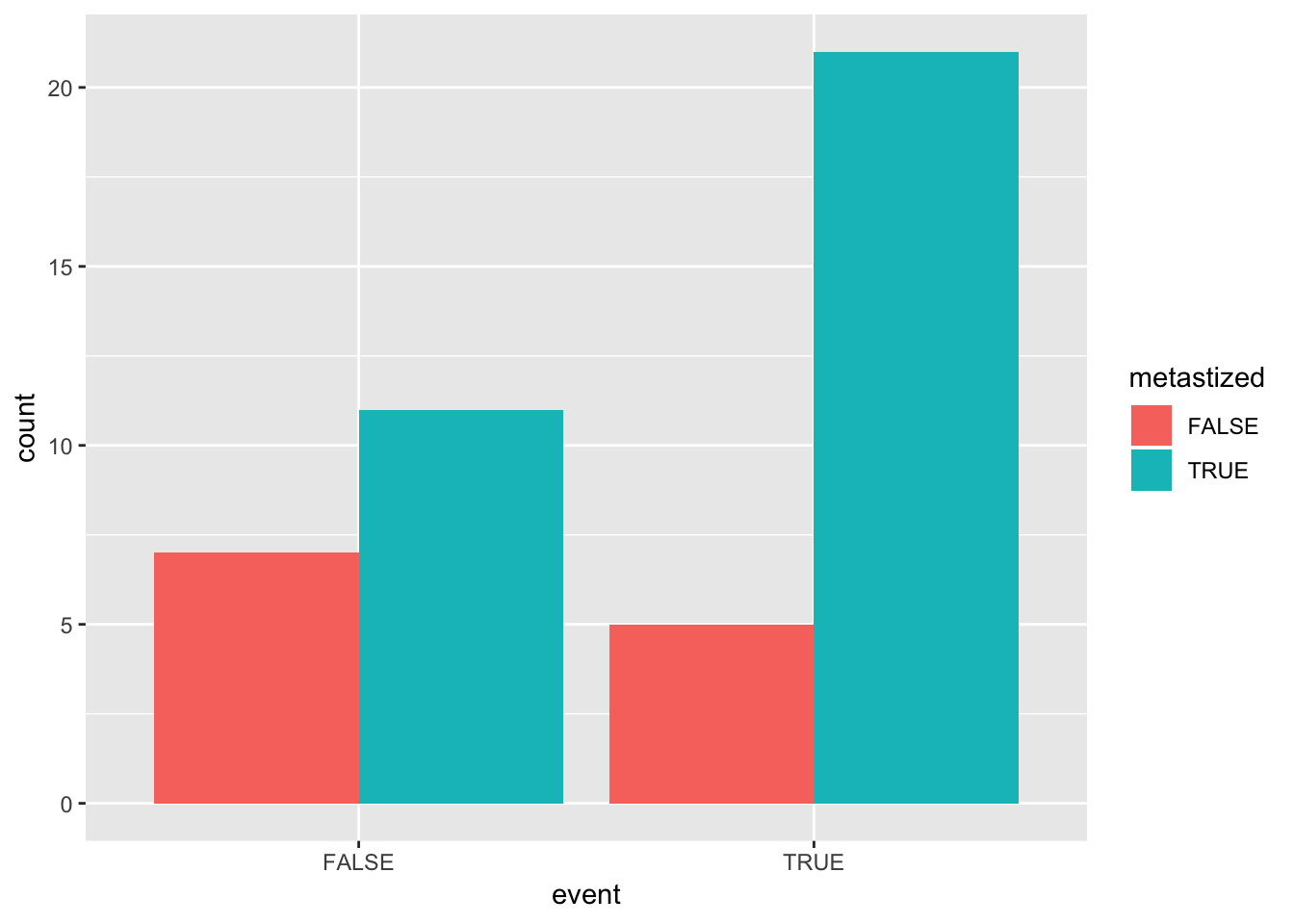## (Censored) Event times

Let’s inspect some of the “global” characteristics of the event time distribution, stratified w.r.t. censoring, i.e. event, and metastization, i.e. metastized.

Stratified quantitative characteristics of event times

event metastized mean_time median_time sd_time
FALSE FALSE 159.0000 198 65.47519
FALSE TRUE 151.0909 145 52.67344
TRUE FALSE 93.6000 69 67.74806
TRUE TRUE 48.0000 40 37.75315

### Histograms

Below we show histograms for each, the event times as well as the censored survival times. The dotted vertical lines show the corresponding means.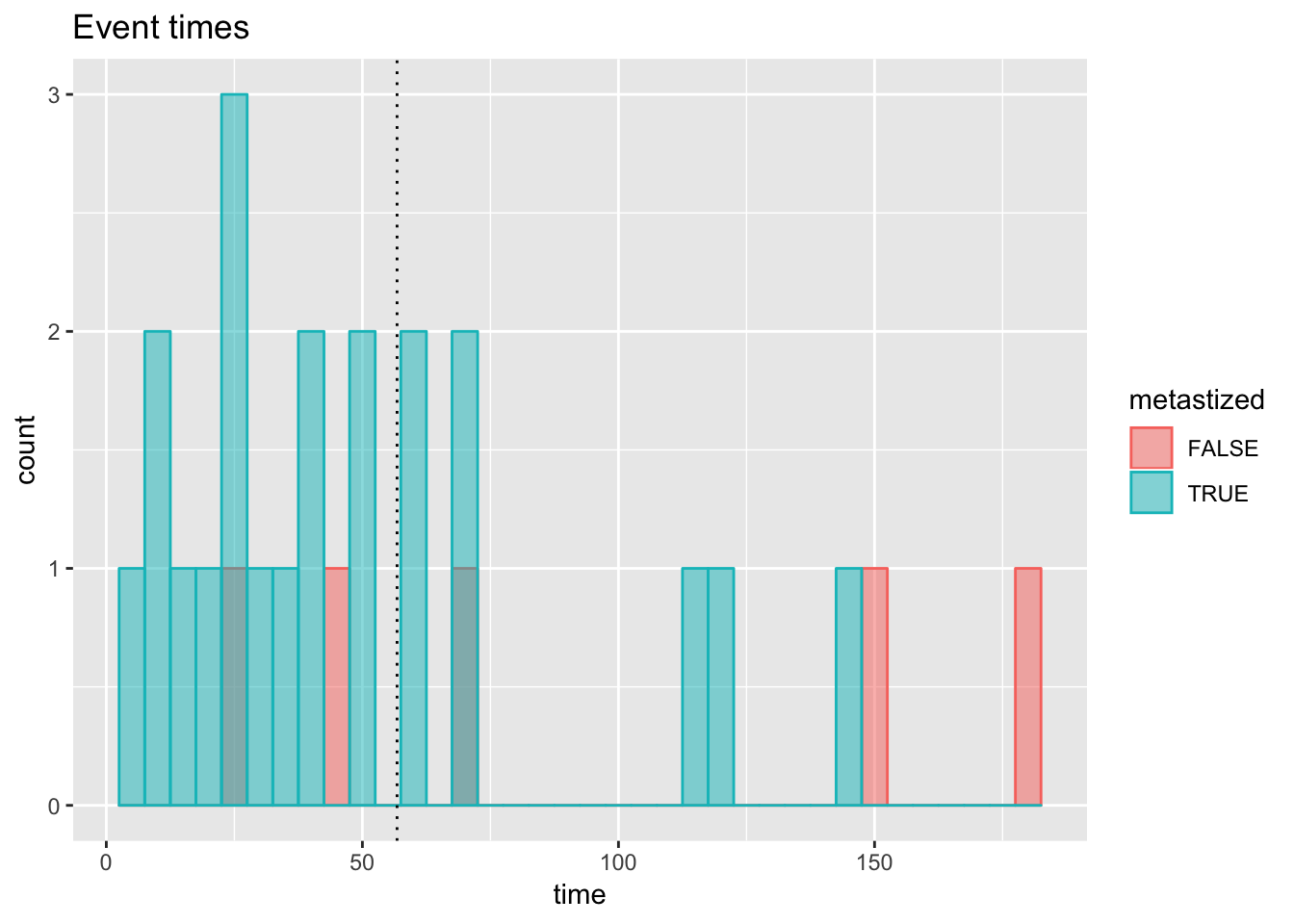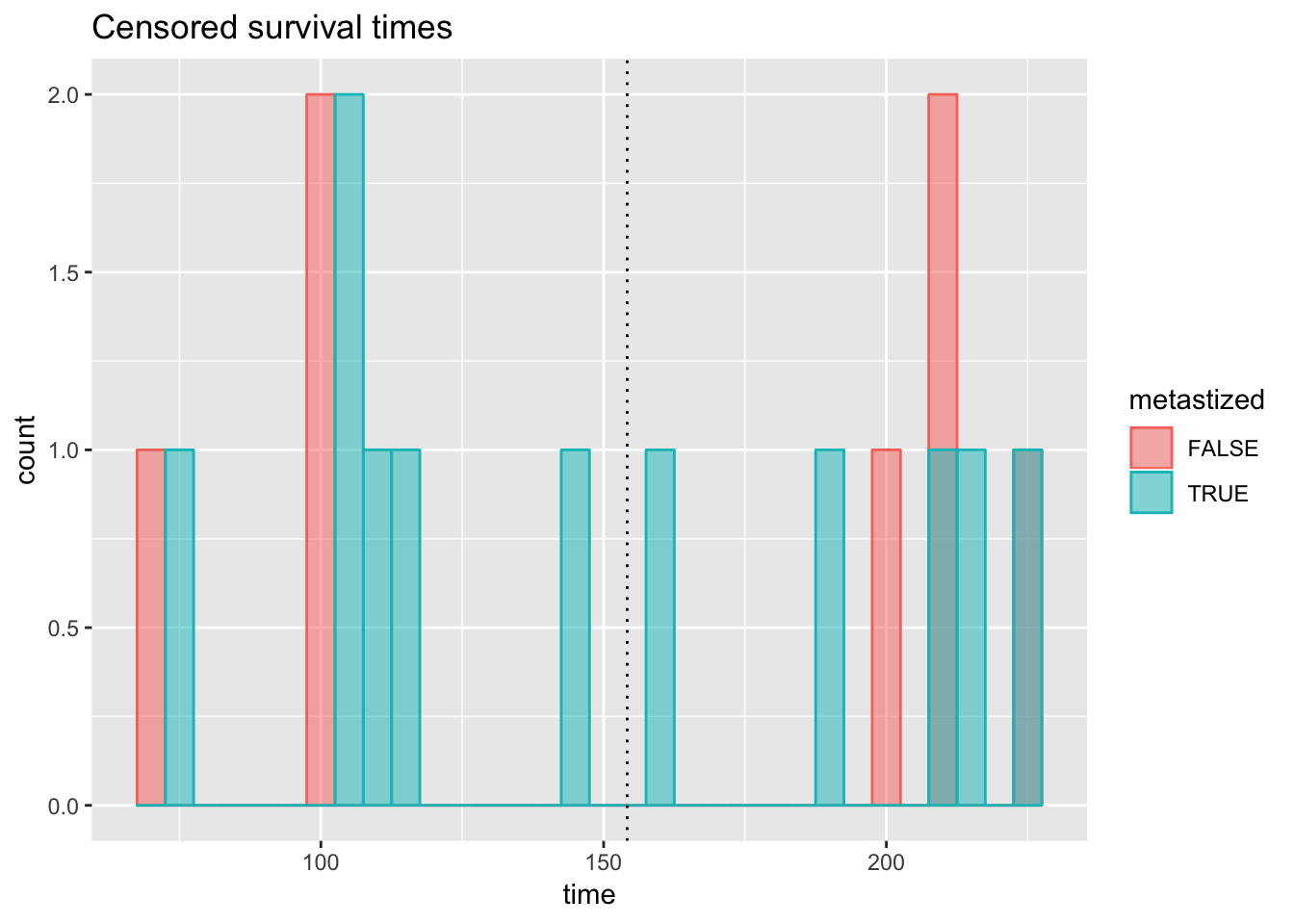# Some math (some notation)

Central to survival models is the survival function $$S(t)$$ defined as

$S(t) = \mathbb{P}[T>t] = e^{-H(t)}$ Here $$T$$ is a the survival time of an individual and thus $$T>t$$ denotes the event that the patient or individual survived beyond time $$t$$. $$H(t)$$ is known as the cumulative hazard and can be shown to be given by

$H(t) = \int_{0}^{t}{\rm d}u~h(u)$

Here we introduced4 We discard the dependence on latent parameters such as $$\mathbf{\beta}$$ or $$\gamma$$ below, for the sake of readability. the hazard rate $$h(t)$$

$h(t;\mathbf{x}) = h_0(t) e^{\mathbf{x}'\cdot\boldsymbol{\beta}}$

Here $$\mathbf{x}$$ is a vector of covariates describing an individual5 In our mastectomy dataset it is simply a scalar indicator corresponding to the column metastized above.. The above makes it apparent why such models are often referred to as proportional hazard models. Further, we make the assumption that the baseline hazard $$h_0$$ fulfills

$h_0(t) = h_0.$

Our Bayesian analysis therefore has the unknown parameters $$\boldsymbol{\beta}$$ and $$h_0$$ where we parametrize the latter as $$h_0 = e^\gamma$$. Note that the above implies (or is equivalent to) $$T$$ having an exponential law with rate parameter equal to $$\exp{(\mathbf{x}'\cdot \boldsymbol{\beta}+ \gamma)}$$.

For the keen reader, try to verify (or convince yourself) that one has in the limit $$dt\rightarrow 0$$

$h(t)dt \doteq \mathbb{P}\left[T\in (t,t+dt) \vert T\geq t\right]$

To come back to our dataset above, we are going use the indicator metastized as the only covariate in $$\mathbf{x}$$ per individual, essentially giving us two baseline hazards for the two sub-populations. More precisely women without metastization prior to surgery are characterised by a (constant) baseline hazard equal to $$\lambda_0=e^{\gamma}$$ and women with metastization prior to surgery are characterised by a (constant) baseline hazard equal to $$e^{\gamma + \beta}$$, which, depending on the sign of $$\beta$$, might be larger or smaller than $$\lambda_0$$.

# Stan

Now let’s get our hands dirty (or actually our keyboard) and start specifying our corresponding generative model in Stan!

## Data block

Here we define precisely the type and dimensions of data provided externally6 E.g. via rstan, pystan or cmdstan. to Stan.

data {
int<lower=1> N_uncensored;
int<lower=1> N_censored;
int<lower=0> NC;
matrix[N_censored,NC] X_censored;
matrix[N_uncensored,NC] X_uncensored;
vector<lower=0>[N_censored] times_censored;
vector<lower=0>[N_uncensored] times_uncensored;
}

N_uncensored and N_censored are the number of women for which event=1 and event=0, respectively. In survival model terminology, the former are uncensored instances, for which death (the event or endpoint of interest) was observed, and the latter are censored instances, for which no event was observed in the observation time time. The variable N_C is the number of covariates, in our case equal to $$1$$, since we only use metastized. Note that we allow for N_C to be $$0$$, which corresponds to the case where we fit one baseline hazard to the entire population.

For the sake of performance7 That is to be able to use vectorized statements, see below we split the actual design matrix into two, corresponding to event=1 and event=0. X_uncensored and X_censored in our particular case will be matrices with only one column, each.

Lastly, times_censored and times_uncensored contain the values of time in the dataframe df, separated according to event=0 and event=1, respectively.

## Parameters block

Here we define all parameters that we wish to infer.

parameters {
vector[NC] betas;
real intercept;
}

Note that betas corresponds to $$\boldsymbol{\beta}$$ and intercept to $$\gamma$$.

## Model block

Here we define the likelihood and priors. Before we do so, I’d like to quote Jonah Gabry:

“Choosing priors is about including information while allowing the chance of being wrong.”

In this sense, let’s hack the model block:

model {
betas ~ normal(0,2);
intercept ~ normal(-5,2);
target += exponential_lpdf(times_uncensored | exp(intercept+X_uncensored*betas));
target += exponential_lccdf(times_censored | exp(intercept+X_censored*betas));
}

To get an intuition for the prior choice of intercept or actually $$\gamma$$, observe that $$e^{-\gamma}$$ is equal to the mean of baseline exponential (which in the data is around $$100$$, hence $$\gamma\approx -4.6$$).

Note that implicit here is the assumption that survival times are mutually independent.

Moreover, above we use vectorized statements, which makes the computation more efficient than using, say, a for loop and iterating over all individuals. This is the main reason why we decided to work with the _censored and _uncensored suffixes and split the data, as opposed to the variant where one keeps the data together and provides an boolean array/vector specifying which patients have a (right-) censored survival time.

## Generated Quantities Block

For the posterior predictive checks we will conduct below, that allow us to scrutinize aspects of our posterior induced family8 Here we adopt the viewpoint that Bayesian statistics leads to families of models, each model weighted approximately proportional to the corresponding posterior probability of it. of survival models, we need to be able to sample survival times, for each individual (or a suitable subset of them), at a set of representative posterior induced model instances. These survival times are stored below in the vector times_uncensored_sampled. Note that we only generate survival times for individuals for which we actually observed an event.

generated quantities {
vector[N_uncensored] times_uncensored_sampled;
for(i in 1:N_uncensored) {
times_uncensored_sampled[i] = exponential_rng(exp(intercept+X_uncensored[i,]*betas));
}
}

A great improvement in Stan $$2.18$$ is the support of vectorized _rng statements, i.e. the possibility to draw vectors of random samples, instead of generating them on scalar based within a for-loop.

## Model compilation

library(rstan)
rstan_options(auto_write = TRUE)
sm <- stan_model("~/Desktop/Stan/A_Survival_Model_in_Stan/exponential_survival_simple_ppc.stan")

# Data preparation

N <- nrow(df)
X <- as.matrix(pull(df, metastized))
is_censored <- pull(df,event)==0
times <- pull(df,time)
msk_censored <- is_censored == 1
N_censored <- sum(msk_censored)

Combine (couple) all the data into one named list with reference names corresponding precisely to the actual names as defined in the data block of our Stan model.

stan_data <- list(N_uncensored=N-N_censored,
N_censored=N_censored,
X_censored=as.matrix(X[msk_censored,]),
X_uncensored=as.matrix(X[!msk_censored,]),
times_censored=times[msk_censored],
times_uncensored = times[!msk_censored],
NC=ncol(X)
)

# Fitting the model

fit <- sampling(sm, data=stan_data, seed=42, chains=4, cores=2, iter=4000)

# Inspecting results

Consider especially the ess and rhat columns below, which correspond to the effective sample size and the potential scale reduction statistics. In a nutshell, rhat should be very close to $$1$$ which indicates that the chain(s) mixed (converged) and ess should be as close as possible to the total number of MCMC iterations, excluding warmup9 Starting in Stan 2.18 ess can in fact be larger than the number of MCMC iterations, essentially due to what is known as anti-correlations (yes NUTS and HMC can sometimes be unbelievable super-efficient!). For more details on the two quantities see the section General MCMC diagnostics in the bayesplot vignette Visual MCMC diagnostics using the bayesplot package. For a detailed example regarding the updates on ess and rhat in 2.18 I can highly recommend Aki Vehtari’s Rank-normalized split-Rhat and relative efficiency estimates (excluding burn-in).

Posterior summary

term estimate std.error conf.low conf.high rhat ess
intercept -5.7456285 0.4259313 -6.6556501 -4.992377 1.001476 1669
betas 0.8702448 0.4787253 -0.0027207 1.858607 1.001180 1761
## 0 of 8000 iterations ended with a divergence.
## 0 of 8000 iterations saturated the maximum tree depth of 10.

## Visual inspection of the posterior

library(bayesplot)
library(survival)
post <- as.array(fit)
fit_cox <- coxph(Surv(time, event)~metastized, data=df)
coef_cox <- coef(fit_cox)
se_cox <- sqrt(fit_cox\$var)

### Kernel density plots of posterior draws with chains separated but overlaid on a single plot.

mcmc_dens_overlay(post, pars=c("betas", "intercept"))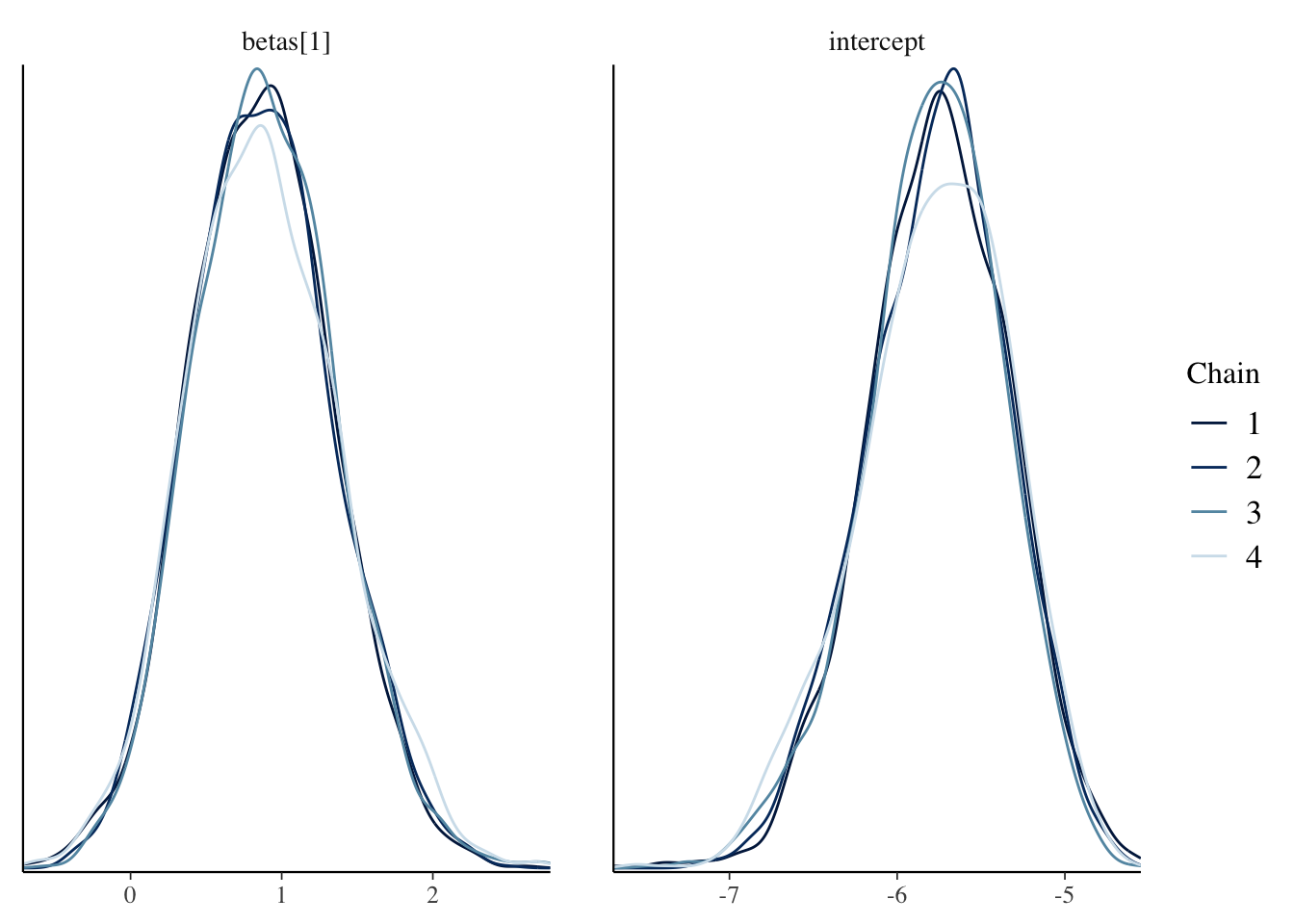### Plots of uncertainty intervals computed from posterior draws with all chains merged.

The three dashed vertical lines below (from left to right) correspond to the frequentist’s10 Using the coxph routine in the survival package, see the code of this RMarkdown. point estimate minus the standard error, the point estimate and the point estimate plus the standard error of the regression coefficient, respectively.

mcmc_intervals(post, pars=c("betas", "intercept")) +
vline_at(c(coef_cox-1.96*se_cox, coef_cox, coef_cox+1.96*se_cox),linetype="dashed")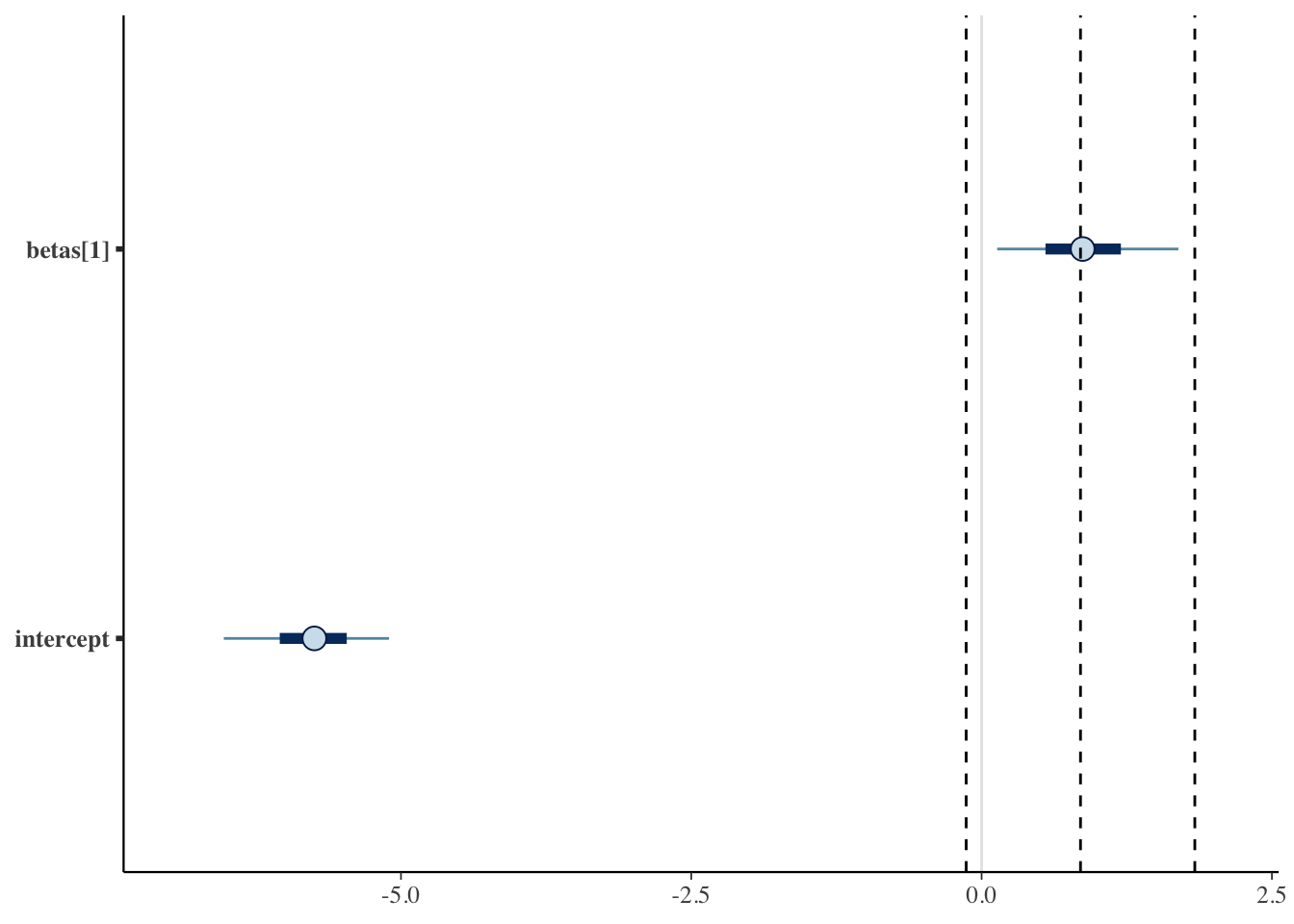### Hazard

Let us also separately compare the 95% credible interval for the baseline (that is metastized==0) hazard with what one would obtain from an exact maximum likelihood calculation:

df_0 <- filter(df, metastized==0)
df_1 <- filter(df_0, event==TRUE)
baseline_hazard_mle <- nrow(df_1)/sum(pull(df_0, "time"))
baseline_hazard_mle_sd <-  sqrt(nrow(df_1))/sum(pull(df_0, "time"))
df_fit <- as.tibble(as.data.frame(fit)) %>% mutate(hazard0=exp(intercept))
mcmc_intervals(df_fit, pars=c("hazard0")) +
vline_at(c(baseline_hazard_mle-1.96*baseline_hazard_mle_sd, baseline_hazard_mle,baseline_hazard_mle+1.96*baseline_hazard_mle_sd),linetype="dashed")+
xlim(0, .015)